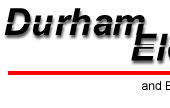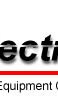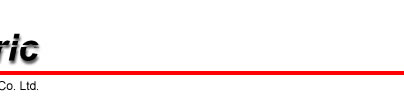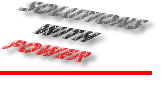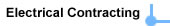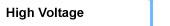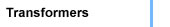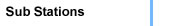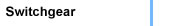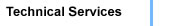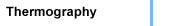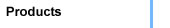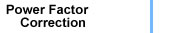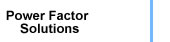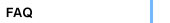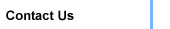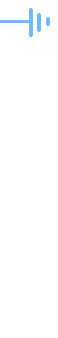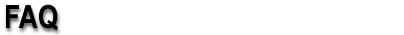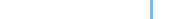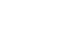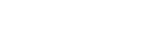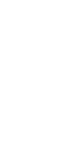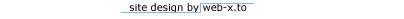## Useful Formulae

Ohms = Volts ÷ Amperes
Amperes = Volts ÷ Ohms
Volts = Amperes x Ohms

### POWER - A.C. CIRCUITS

Power Factor = Watts ÷ (Volts Amperes)
Three Phase Kilowatts = (Volts x Amperes x Power Factor x 1.732) ÷ 1000
Three Phase Volt - Amperes = Volts x Amperes x 1.732
Three Phase Amperes = (746 x Horsepower) ÷ (1.732 x Volts x Efficiency x Power Factor)
Single Phase Kilowatts = (Volts x Amperes x Power Factor) ÷ 1000
Single Phase Amperes = (746 x Horsepower) ÷ (Volts x Efficiency x Power Factor)

### POWER - D.C. CIRCUITS

Watts = Volts x Amperes
Amperes = Watts ÷ Volts
Horsepower (Volts x Amperes x Efficiency) ÷ 746

### MOTOR APPLICATION FORMULAS

Torque (lbs. - ft.) = (Horsepower x 5250) ÷ RPM
Shaft Stress (lbs. per sq. inch) = (Horsepower x 321,000) ÷ (RPM x Shaft Diam³.)

### FOR PUMPS

Horsepower = (GPM x Head in Feet x Specific Gravity) ÷ (3960 x Efficiency)

### FOR FANS & BLOWERS

Horsepower = (CFM x Pressure (lbs. per sq. ft.)) ÷ (33000 x Efficiency)

### SPEED

Synchronous RPM = (Hertz x 120) ÷ Poles
Percent Slip = (Synchronous RPM - Full Load RPM) ÷ Synchronous RPM x 100